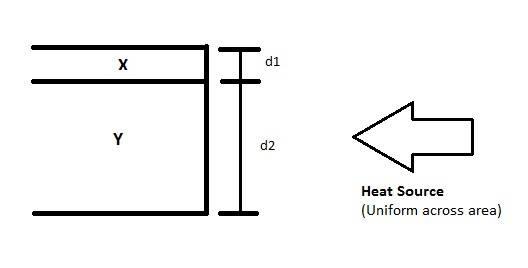# Thermal Conductivites between two dissimilar metals1. Homework Statement

I've been failing to find examples dealing with "longitudinal" thermal conductivity and how two objects in contact with each other would affect the heat flow compared to not being in contact at all.

Problem: Given the following information, determine the thermal energy at 1 meter from the heat source for material X for the two cases 1) Where material X is not contact with material Y 2) Where material X and Y are in contact as shown. For both cases, first assume materials are in vacuum, then assume there are in ambient air at STP (273 K).

## Homework Equations

Thermal Conductivity
X: 2,000 W/(m K)
Y: 50 W/(m K)

d1 = 0.00001 m
d2 = 1 m

Heat Source
q = 100 W

Heat Transfer Coefficient
Air: 10 W/(m^2 K)

## The Attempt at a Solution

What I am struggling with is whether the conduction between X and Y would essentially "shorten" the conduction of material X itself. I am also unsure if thermal radiation would come into play. Any help is appreciated!